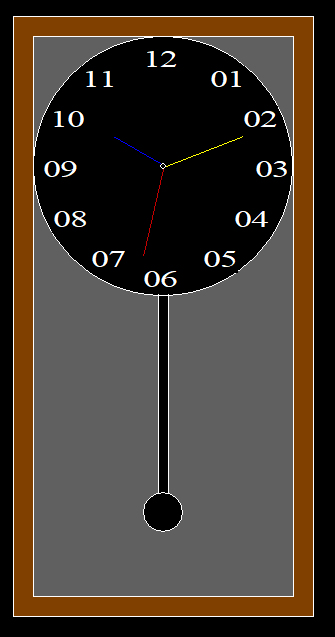# C program to create a pendulum clock using graphics

• Last Updated : 23 Apr, 2021

In this article, it is discussed how to design a Pendulum Clock in the C programming language using Graphics.

Approach:

• Create two rectangles, one inside the other, using the rectangle() function to act as the outer outline of the clock & the other is the inner outline of the clock.
• Color the space between the two rectangles brown using setfillstyle() and floodfill() functions
• Implement a circle inside the inner rectangle using the circle() function
• Color all other parts leaving the circle with dark gray using setfillstyle() and floodfill() functions.
• In the circle, insert all the digits using settextstyle() and outtextxy() functions.
• Calculate the coordinates of the digits.
• Implement the pendulum with two lines by using the line() function & another circle by using the circle() function which will act as the bob.
• Color all of them black using the setfillstyle() and floodfill() functions again.
• Implement hour, minute & second hands by using the line() function.
• Color the objects individually by using the setcolor() function.

Below is the implementation of the above approach:

## C

 `// C program toc draw the pendulum clock``#include ``#include ``#include `` ` `// Driver Code``void` `main()``{``    ``int` `gd = DETECT, gm;`` ` `    ``// Initialize of gdriver``    ``initgraph(&gd, &gm, ``"C:\\"``                        ``"turboc3\\bgi"``);`` ` `    ``// Clock Outer Outline``    ``rectangle(500, 50, 800, 650);`` ` `    ``// Clock Inner Outline``    ``rectangle(520, 70, 780, 630);`` ` `    ``// Coloring Middle Part Of``    ``// Rectangle With Brown``    ``setfillstyle(SOLID_FILL, BROWN);``    ``floodfill(505, 55, 15);`` ` `    ``// Clock Outline``    ``circle(650, 200, 130);``    ``circle(650, 200, 3);`` ` `    ``// Coloring all the parts Of the``    ``// clock except the circle with``    ``// Darkgray``    ``setfillstyle(SOLID_FILL, DARKGRAY);``    ``floodfill(525, 355, 15);``    ``floodfill(522, 72, 15);``    ``floodfill(768, 72, 15);`` ` `    ``// Inserting Digits``    ``settextstyle(6, 0, 3);``    ``outtextxy(697, 100, ``"01"``);``    ``outtextxy(730, 140, ``"02"``);``    ``outtextxy(742, 190, ``"03"``);``    ``outtextxy(721, 240, ``"04"``);``    ``outtextxy(690, 280, ``"05"``);``    ``outtextxy(630, 300, ``"06"``);``    ``outtextxy(578, 280, ``"07"``);``    ``outtextxy(540, 240, ``"08"``);``    ``outtextxy(530, 190, ``"09"``);``    ``outtextxy(537, 140, ``"10"``);``    ``outtextxy(569, 100, ``"11"``);``    ``outtextxy(630, 80, ``"12"``);`` ` `    ``// Left Line Of Pendulum``    ``line(645, 328, 645, 528);`` ` `    ``// Right Line Of Pendulum``    ``line(655, 328, 655, 528);`` ` `    ``// Pendulum Bob``    ``circle(650, 546, 20);`` ` `    ``// Coloring Line & Bob With Black``    ``setfillstyle(SOLID_FILL, BLACK);``    ``floodfill(652, 544, 15);``    ``floodfill(647, 330, 15);`` ` `    ``// Creating the Hour Hand``    ``// & Color Blue``    ``setcolor(BLUE);``    ``line(647, 197, 600, 170);`` ` `    ``// Creating Minute Hand``    ``// & Color Yellow``    ``setcolor(YELLOW);``    ``line(653, 200, 730, 170);`` ` `    ``// Creating Second Hand and the``    ``// Color Red``    ``setcolor(RED);``    ``line(650, 203, 630, 290);`` ` `    ``// Hold the screen for a while``    ``getch();`` ` `    ``// Close the initialized gdriver``    ``closegraph();``}`

Output:My Personal Notes arrow_drop_up